Filter by type:

Hyper Natural Deduction for Gödel Logic — natural deduction system for parallel reasoning

journal
Arnold Beckmann and Norbert Preining
Journal Logic Computation, Volume 28, Issue 6, September 2018, Pages 1125–1187.
Publication year: 2018

We introduce a system of Hyper Natural Deduction for Gödel Logic as an extension of Gentzen’s system of Natural Deduction. A deduction in this system consists of a finite set of derivations which uses the typical rules of Natural Deduction, plus additional rules providing means for communication between derivations. We show that our system is sound and complete for infinite-valued propositional Gödel Logic, by giving translations to and from Avron’s Hypersequent Calculus. We provide conversions for normalization extending usual conversions for Natural Deduction and prove the existence of normal forms for Hyper Natural Deduction for Gödel Logic. We show that normal deductions satisfy the subformula property.

Feasible set functions have small circuits

feasible set fctsjournal
Arnold Beckmann, Samuel R. Buss, Sy-David Friedman, Moritz Müller and Neil Thapen
Computability, Volume 8, Number 1, Pages 67 - 98.
Publication year: 2018

The Cobham Recursive Set Functions (CRSF) provide an analogue of polynomial time computation which applies to arbitrary sets. We give three new equivalent characterizations of CRSF. The first is algebraic, using subset-bounded recursion and a form of Mostowski collapse. The second is our main result: the CRSF functions are shown to be precisely the functions computed by a class of uniform, infinitary, Boolean circuits. The third is in terms of a simple extension of the rudimentary functions by transitive closure and subset-bounded recursion.

Total Search Problems in Bounded Arithmetic and Improved Witnessing

conference
Arnold Beckmann and Jean-Jose Razafindrakoto
24th International Workshop on Logic, Language, Information, and Computation (WoLLiC), 2017. Pages: 31 - 47
Publication year: 2017

We define a new class of total search problems as a subclass of Megiddo and Papadimitriou’s class of total $\NP$ search problems, in which solutions are verifiable in $\AC^0$. We denote this class $\forall\exists\AC^0$. We show that all total $\NP$ search problems are equivalent wrt. $\AC^0$-many-one reductions to search problems in $\forall\exists\AC^0$. Furthermore, we show that $\forall\exists\AC^0$ contains well-known problems such as the Stable Marriage and the Maximal Independent Set problems. We introduce the class of Inflationary Iteration problems in $\forall\exists\AC^0$ and show that it characterizes the provably total $\NP$ search problems of the bounded arithmetic theory corresponding to polynomial-time. Cook and Nguyen introduced a generic way of defining a bounded arithmetic theory $\VC$ for complexity classes $\C$ which can be obtained using a complete problem. For such $C$ we will define a new class $\KPT[C]$ of $\forall\exists\AC^0$ search problems based on Student-Teacher games in which the student has computing power limited to $\AC^0$. We prove that $\KPT[C]$ characterizes the provably total $\NP$ search problems of the bounded arithmetic theory corresponding to $\C$. All our characterizations are obtained via “new-style” witnessing theorems, where reductions are provable in a theory corresponding to $\AC^0$.

The NP Search Problems of Frege and Extended Frege Proofs

journal
Arnold Beckmann, Samuel R Buss
ACM TOCL 2017, 18(2): 11:1-11:19
Publication year: 2017

We study consistency search problems for Frege and extended Frege proofs, namely the NP search problems of finding syntactic errors in Frege and extended Frege proofs of contradictions. The input is a polynomial time function, or an oracle, describing a proof of a contradiction; the output is the location of a syntactic error in the proof. The consistency search problems for Frege and extended Frege systems are shown to be many-one complete for the provably total NP search problems of the second order bounded arithmetic theories U^1_2 and V^1_2 , respectively.

On Transformations of Constant Depth Propositional Proofs

preprint
Arnold Beckmann and Sam Buss
Publication year: 2017

This paper studies the complexity of constant depth propositional proofs in the cedent and sequent calculus. We discuss the relationships between the size of tree-like proofs, the size of dag-like proofs, and the heights of proofs. The main result is to correct a proof construction in an earlier paper about transformations from proofs with polylogarithmic height and constantly many formulas per cedent.

Deciding logics of linear Kripke frames with scattered end pieces

journal
Arnold Beckmann and Norbert Preining
Soft Computing 2017, 21(1): 191-197
Publication year: 2017

We show that logics based on linear Kripke frames – with or without constant domains – that have a scattered end piece are not recursively enumerable. This is done by reduction to validity in all finite classical models.

Cobham Recursive Set Functions and Weak Set Theories

feasible set fctsjournal
Arnold Beckmann, Samuel R. Buss, Sy-David Friedman, Moritz Müller and Neil Thapen
Lecture Notes Series, Institute for Mathematical Sciences, National University of Singapore: Volume 33, 2017, pp. 55-116
Publication year: 2017

This paper introduces bounded fragments of Kripke Platek set theory which characterise the Cobham Recursive Set Functions.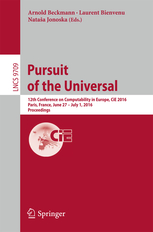Pursuit of the Universal

edited volumes
Arnold Beckmann, Laurent Bienvenu, Nataša Jonoska
12th Conference on Computability in Europe, CiE 2016, Paris, France, June 27 - July 1, 2016, Proceedings, LNCS 9709
Publication year: 2016

Cobham Recursive Set Functions

feasible set fctsjournal
Arnold Beckmann, Samuel R. Buss, Sy-David Friedman, Moritz Müller and Neil Thapen
APAL 2016, 167(3): 335-369
Publication year: 2016

This paper introduces the Cobham Recursive Set Functions (CRSF) as a version of polynomial time computable functions on general sets, based on a limited (bounded) form of epsilon-recursion. This is inspired by Cobham’s classic definition of polynomial time functions based on limited recursion on notation. We introduce a new set composition function, and a new smash function for sets which allows polynomial increases in the ranks and in the cardinalities of transitive closures. We bootstrap CRSF, prove closure under (unbounded) replacement, and prove that any CRSF function is embeddable into a smash term. When restricted to natural encodings of binary strings as hereditarily finite sets, the CRSF functions define precisely the polynomial time computable functions on binary strings. Prior work of Beckmann, Buss and Friedman and of Arai introduced set functions based on safe-normal recursion in the sense of Bellantoni-Cook. We prove an equivalence between our class CRSF and a variant of Arai’s predicatively computable set functions.

Separating intermediate predicate logics of well-founded and dually well-founded structures by monadic sentences

journal
Arnold Beckmann and Norbert Preining
Journal Logic Computation (2015) 25(3): 527-547
Publication year: 2015

We consider intermediate predicate logics defined by fixed well-ordered (or dually well-ordered) linear Kripke frames with constant domains where the order-type of the well-order is strictly smaller than~$\omega^\omega$. We show that two such logics of different order-type are separated by a first-order sentence using only one monadic predicate symbol. Previous results by Minari, Takano and Ono, as well as the second author, obtained the same separation but relied on the use of predicate symbols of unbounded arity.

Safe Recursive Set Functions

feasible set fctsjournal
Arnold Beckmann, Samuel R. Buss and Sy-David Friedman
JSL 2015, 80(3): 730-762
Publication year: 2015

We introduce the safe recursive set functions based on a Bellantoni-Cook style subclass of the primitive recursive set functions. We show that the functions computed by safe recursive set functions under a list encoding of finite strings by hereditarily finite sets are exactly the polynomial growth rate functions computed by alternating exponential time Turing machines with polynomially many alternations. We also show that the functions computed by safe recursive set functions under a more efficient binary tree encoding of finite strings by hereditarily finite sets are exactly the quasipolynomial growth rate functions computed by alternating quasipolynomial time Turing machines with polylogarithmic many alternations.
We characterize the safe recursive set functions on arbitrary sets in definability-theoretic terms. In its strongest form, we show that a function on arbitrary sets is safe recursive if, and only if, it is uniformly definable in some polynomial level of a refinement of Jensen’s J-hierarchy, relativised to the transitive closure of the function’s arguments.
We observe that safe-recursive functions on infinite binary strings are equivalent to functions computed by so-called infinite-time Turing machines in time less than ω^ω. We also give a machine model for safe recursion which is based on set-indexed parallel processors and the natural bound on running times.

Hyper Natural Deduction

conference
Arnold Beckmann and Norbert Preining
Symposium on Logic in Computer Science (LICS), 2015, 30th Annual ACM/IEEE. Pages: 547 - 558
Publication year: 2015

We introduce a Hyper Natural Deduction system as an extension of Gentzen’s Natural Deduction system. A Hyper Natural Deduction consists of a finite set of derivations which may use, beside typical Natural Deduction rules, additional rules providing means for communication between derivations. We show that our Hyper Natural Deduction system is sound and complete for infinite-valued propositional Gödel Logic, by giving translations to and from Avron’s Hyper sequent Calculus. We also provide conversions for normalisation and prove the existence of normal forms for our Hyper Natural Deduction system.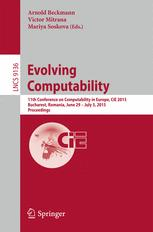Evolving Computability

edited volumes
Arnold Beckmann, Victor Mitrana, Mariya Soskova
11th Conference on Computability in Europe, CiE 2015, Bucharest, Romania, June 29-July 3, 2015. Proceedings, LNCS9136
Publication year: 2015Selected papers of the "Turing Centenary Conference: CiE 2012"

special issues
Arnold Beckmann, Anuj Dawar
special issue of the journal Logical Methods in Computer Science,
Publication year: 2014

Parity Games and Propositional Proofs

journal
Arnold Beckmann, Pavel Pudlák and Neil Thapen
ACM TOCL 2014, 15(2): 17:1-17:30
Publication year: 2014

A propositional proof system is weakly automatizable if there is a polynomial time algorithm which separates satisfiable formulas from formulas which have a short refutation in the system, with respect to a given length bound. We show that if the resolution proof system is weakly automatizable, then parity games can be decided in polynomial time. We give simple proofs that the same holds for depth-1 propositional calculus (where resolution has depth 0) with respect to mean payoff and simple stochastic games. We define a new type of combinatorial game and prove that resolution is weakly automatizable if and only if one can separate, by a set decidable in polynomial time, the games in which the first player has a positional winning strategy from the games in which the second player has a positional winning strategy.
Our main technique is to show that a suitable weak bounded arithmetic theory proves that both players in a game cannot simultaneously have a winning strategy, and then to translate this proof into propositional form.Language, Life, Limits

edited volumes
Arnold Beckmann, Erzsébet Csuhaj-Varjú, Klaus Meer
10th Conference on Computability in Europe, CiE 2014, Budapest, Hungary, June 23-27, 2014, Proceedings, LNCS 8493
Publication year: 2014

Improved Witnessing and Local Improvement Principles for Second-Order Bounded Arithmetic

journal
Arnold Beckmann and Samuel R Buss
ACM TOCL 2014, 15(1): 2:1-2:35
Publication year: 2014

This paper concerns the second order systems U^1_2 and V^1_2 of bounded arithmetic, which have proof theoretic strengths corresponding to polynomial space and exponential time computation. We formulate improved witnessing theorems for these two theories by using S^1_2 as a base theory for proving the correctness of the polynomial space or exponential time witnessing functions. We develop the theory of nondeterministic polynomial space computation in U^1_2 . Kołodziejczyk, Nguyen, and Thapen have introduced local improvement properties to characterize the provably total NP functions of these second order theories. We show that the strengths of their local improvement principles over U^1_2 and V^1_2 depend primarily on the topology of the underlying graph, not the number of rounds in the local improvement games. The theory U^1_2 proves the local improvement principle for linear graphs even without restricting to logarithmically many rounds. The local improvement principle for grid graphs with only logarithmically rounds is complete for the provably total NP search problems of V^1_2 . Related results are obtained for local improvement principles with one improvement round, and for local improvement over rectangular grids.

Parity Games and Propositional Proofs

conference
Arnold Beckmann, Pavel Pudlák and Neil Thapen
38th International Symposium on Mathematical Foundations of Computer Science, MFCS 2013, Klosterneuburg, Austria, August 26-30, 2013. LNCS 8087. Pages: 111-122
Publication year: 2013

A propositional proof system is weakly automatizable if there is a polynomial time algorithm which separates satisfiable formulas from formulas which have a short refutation in the system, with respect to a given length bound. We show that if the resolution proof system is weakly automatizable, then parity games can be decided in polynomial time. We also define a combinatorial game and prove that resolution is weakly automatizable if and only if one can separate, by a set decidable in polynomial time, the games in which the first player has a positional winning strategy from the games in which the second player has a positional winning strategy.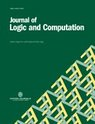Computability in Europe 2009

special issues
Klaus Ambos-Spies, Arnold Beckmann, Erzsebet Csuhaj-Varju, Benedikt Löwe
special issue of the journal Journal of Logic and Computation, Volume 23, Number 4
Publication year: 2013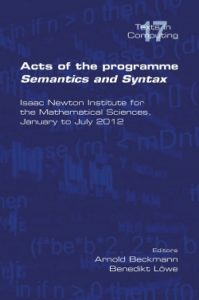Acts of the Programme Semantics and Syntax

edited volumes
Arnold Beckmann and Benedikt Löwe
College Publication, 19 June 2013. ISBN 978-1-84890-080-6.
Publication year: 2013

This volume documents the presentations that were given as part of the research programme Semantics and Syntax: A Legacy of Alan Turing, held at the Isaac Newton Institute for the Mathematical Sciences in Cambridge, UK, 9 January – 6 July 2012.

Using Domain Specific Languages to Support Verification in the Railway Domain

conference
Phillip James, Arnold Beckmann, Markus Roggenbach
Hardware and Software: Verification and Testing. 8th International Haifa Verification Conference, HVC 2012 Haifa, Israel, November 6-8, 2012. Pages: 274 -275
Publication year: 2012

We explore the support of automatic verification via careful design of a domain specific language (DSL) in the context of algebraic specification. Formally a DSL is a loose specification the logical closure of which we regard as implicitly encoded “domain knowledge”. We systematically exploit this “domain knowledge” for automatic verification. We illustrate these ideas within the Railway Domain using the algebraic specification language Casl and an existing DSL, designed by Bjøerner, for modelling railways. Empirical evidence to the benefit of our approach is given in the form of the successful automatic verification of four railway track plans of real world complexity.Computability in Europe 2009

special issues
Klaus Ambos-Spies, Arnold Beckmann, Sam Buss, Benedikt Löwe
special issue of the journal Annals of Pure and Applied Logic, Volume 163, Issue 5
Publication year: 2012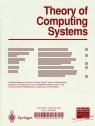Computability in Europe 2009

special issues
Arnold Beckmann, Wolfgang Merkle, Benedikt Löwe
special issue of the journal Theory of Computing Systems, Volume 51, Number 1
Publication year: 2012Computability in Europe 2008

special issues
Arnold Beckmann, Benedikt Löwe
special issue of the journal Journal of Logic and Computation, Volume 22, Number 2
Publication year: 2012

journal
Arnold Beckmann and Sam Buss
TCS 2011, 412(39): 5433-5445
Publication year: 2011

The paper corrects earlier upper bounds on the size of free-cut elimination. Free-cut elimination allows cut elimination to be carried out in the presence of non-logical axioms. The correction requires that the notion of a free-cut be modified so that a cut formula is anchored provided that all of its introductions are anchored, instead of only requiring that one of its introductions is anchored. With the correction, the originally proved size upper bounds remain unchanged. These results also apply to partial cut elimination. We also apply these bounds to elimination of cuts in propositional logic.
If the non-logical inferences are closed under cut and infer only atomic formulas, then all cuts can be eliminated. This generalizes earlier results of Takeuti and of Negri and van Plato.Computability in Europe 2008

special issues
Arnold Beckmann, Benedikt Löwe
special issue of the journal Theory of Computing Systems, Volume 48, Number 3
Publication year: 2011

On the computational complexity of cut-reduction

journal
Klaus T Aehlig and Arnold Beckmann
APAL 2010, 161(6): 711-736
Publication year: 2010

Using appropriate notation systems for proofs, cut-reduction can often be rendered feasible on these notations, and explicit bounds can be given. Developing a suitable notation system for Bounded Arithmetic, and applying these bounds, all the known results on definable functions of certain such theories can be reobtained in a uniform way.Computability in Europe 2008

special issues
Arnold Beckmann, Costas Dimitracopoulos, Benedikt Löwe
special issue of the journal Archive for Mathematical Logic, Volume 49, Number 2
Publication year: 2010

Characterising Definable Search Problems in Bounded Arithmetic via Proof Notations

journal
Arnold Beckmann and Sam Buss
In: Ways of Proof Theory, ed. R. Schindler, Ontos Series on Mathematical Logic, pp. 65-134, 2010.
Publication year: 2010

The complexity class of Π^p_k – Polynomial Local Search (PLS) problems with Π^p_l – goal is introduced, and is used to give new characterisations of definable search problems in fragments of Bounded Arithmetic. The characterisations are established via notations for propositional proofs obtained by translating Bounded Arithmetic proofs using the Paris-Wilkie-translation. For l ≤ i ≤ k , the Σ bi – definable search problems of T^k+1_2 are exactly characterised by Π^p_k – PLS problems with Π^p_l – goals. These Π^p_k – PLS problems can be defined in a weak base theory such as S^1_2 , and proved to be total in T^k+1_2 . Furthermore, the Π^p_k – PLS definitions can be Skolemised with simple polynomial time functions. The Skolemised Π^p_k – PLS definitions give rise to a new ∀Σ^b_1(α) – principle conjectured to separate T^k_2(α) and T^k+1_2(α).

Polynomial Local Search in the Polynomial Hierarchy and Witnessing in Fragments of Bounded Arithmetic

journal
Arnold Beckmann and Sam Buss
Journal of Mathematical Logic 2009, 9(1): 103-138
Publication year: 2009

The complexity class of Π^p_k – polynomial local search (PLS) problems is introduced and is used to give new witnessing theorems for fragments of bounded arithmetic. For 1 ≤ i ≤ k+1 , the Σ^b_i – definable functions of T^k+1_2 are characterized in terms of Π^p_k – PLS problems. These Π^p_k – PLS problems can be defined in a weak base theory such as S^1_2 , and proved to be total in T^k+1_2 . Furthermore, the Π^p_k – PLS definitions can be skolemized with simple polynomial time functions, and the witnessing theorem itself can be formalized, and skolemized, in a weak base theory. We introduce a new ∀Σ^b_1(α) – principle that is conjectured to separate T^k_2(α) and T^k+1_2(α) .